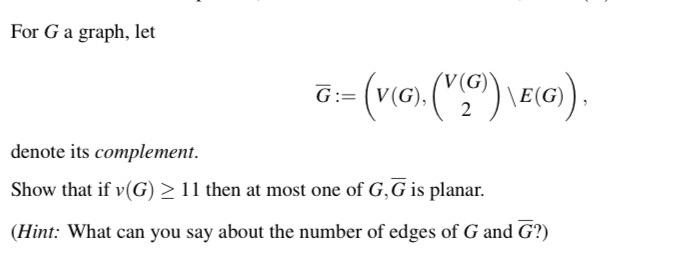Home / Expert Answers / Other Math / for-g-a-graph-let-bar-g-left-v-g-left-begin-array-c-v-g-2-end-array-right-pa888

# (Solved): For $$G$$ a graph, let $\bar{G}:=\left(V(G),\left(\begin{array}{c} V(G) \\ 2 \end{array}\right) ...For $$G$$ a graph, let \[ \bar{G}:=\left(V(G),\left(\begin{array}{c} V(G) \\ 2 \end{array}\right) \backslash E(G)\right)$ denote its complement. Show that if $$v(G) \geq 11$$ then at most one of $$G, \bar{G}$$ is planar. (Hint: What can you say about the number of edges of $$G$$ and $$\bar{G} ?$$ ?)

We have an Answer from Expert